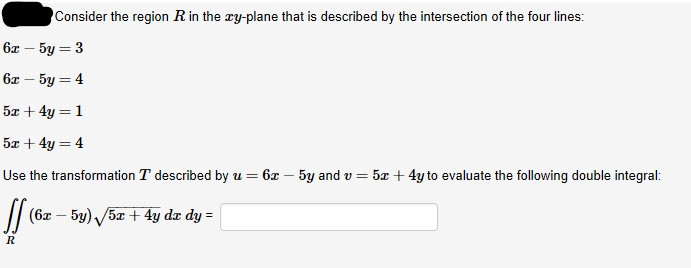# Consider the region R in the ry-plane that is described by the intersection of the four lines:6x – 5y = 36x – 5y = 45x + 4y = 15x + 4y = 45x + 4y to evaluate the following double integral:Use the transformation T described by u = 6r – 5y and v =// (6x – 5y) 5æ+ 4y dæ dy =

Question
1 viewshelp_outlineImage TranscriptioncloseConsider the region R in the ry-plane that is described by the intersection of the four lines: 6x – 5y = 3 6x – 5y = 4 5x + 4y = 1 5x + 4y = 4 5x + 4y to evaluate the following double integral: Use the transformation T described by u = 6r – 5y and v = // (6x – 5y) 5æ+ 4y dæ dy = fullscreen
check_circle

Step 1

### Want to see the full answer?

See Solution

#### Want to see this answer and more?

Solutions are written by subject experts who are available 24/7. Questions are typically answered within 1 hour.*

See Solution
*Response times may vary by subject and question.
Tagged in

### Calculus